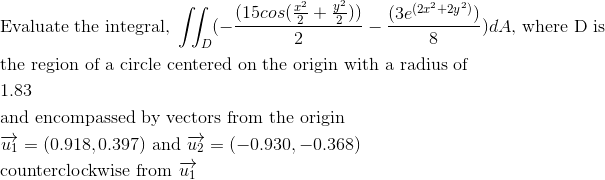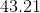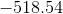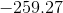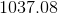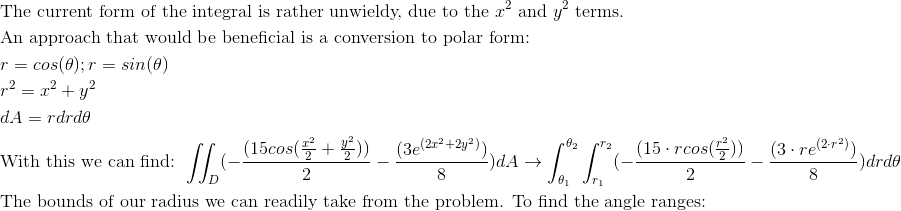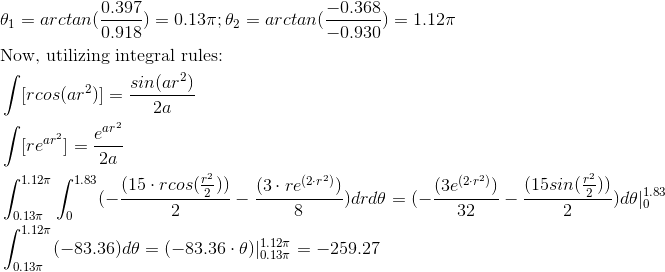Calculus 3 : Double Integrals

Example Questions

Example Question #41 : Double Integration In Polar Coordinates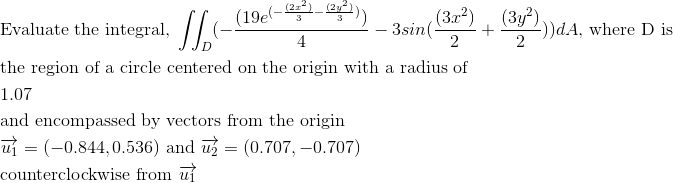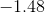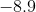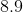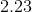Explanation: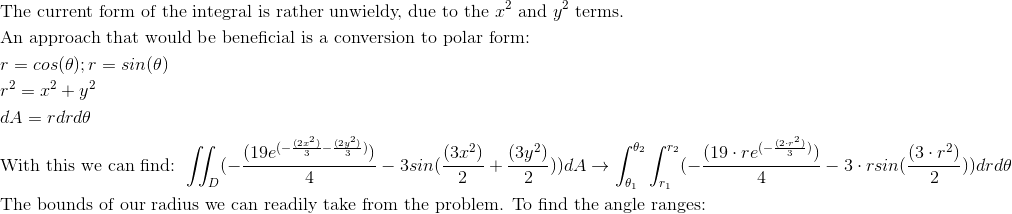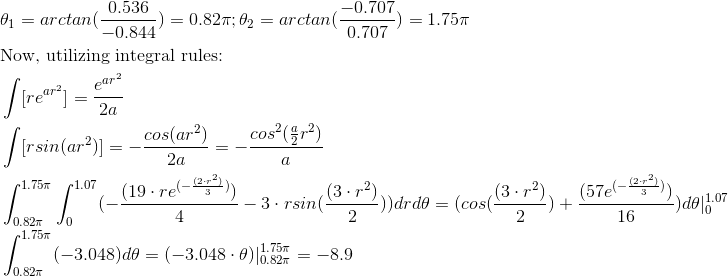Example Question #42 : Double Integration In Polar Coordinates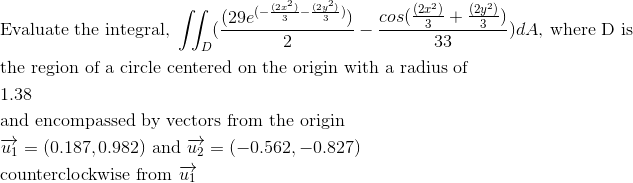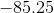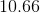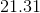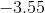Explanation: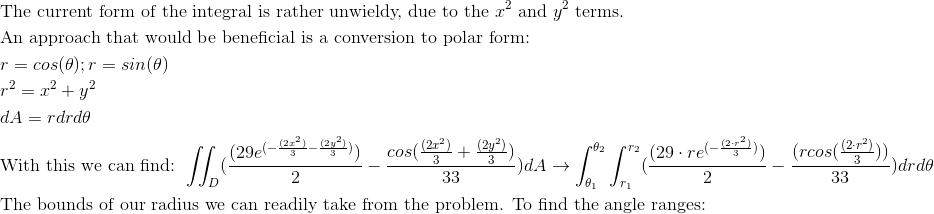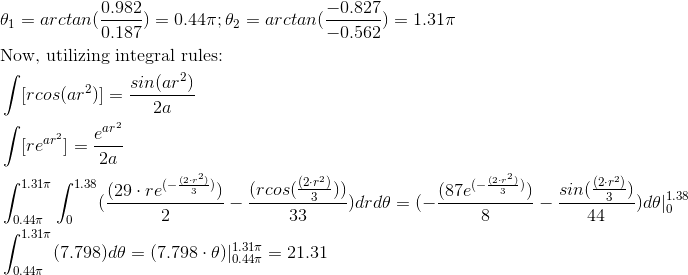Example Question #43 : Double Integration In Polar Coordinates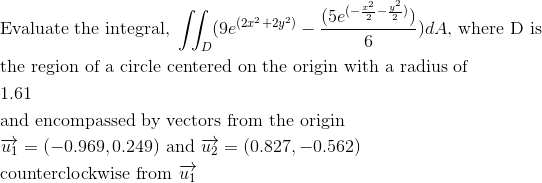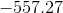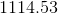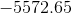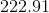Explanation: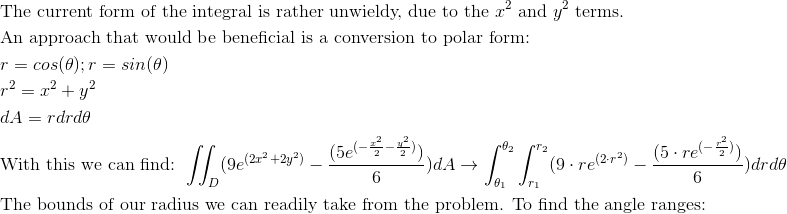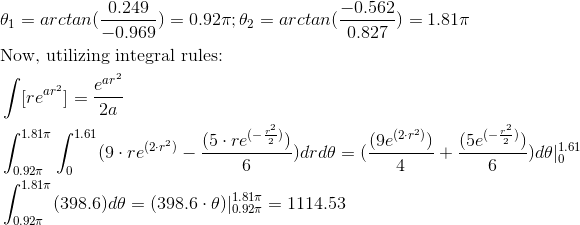Example Question #44 : Double Integration In Polar Coordinates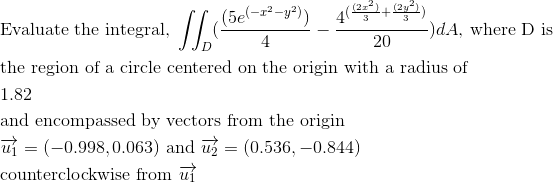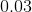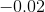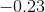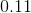Explanation: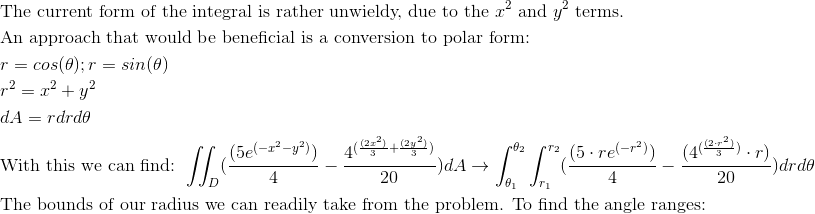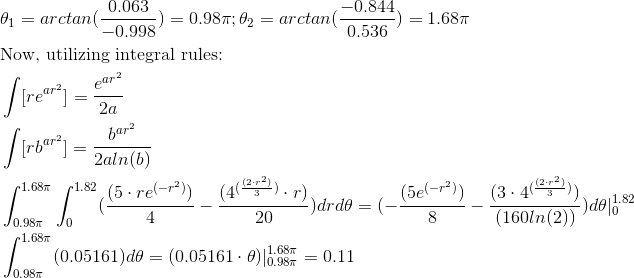Example Question #45 : Double Integration In Polar Coordinates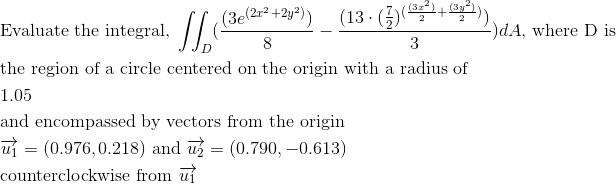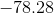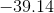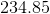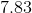Explanation: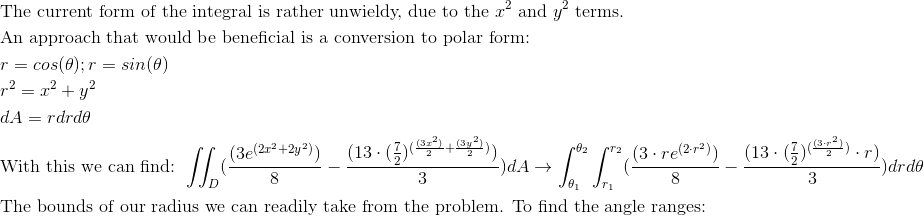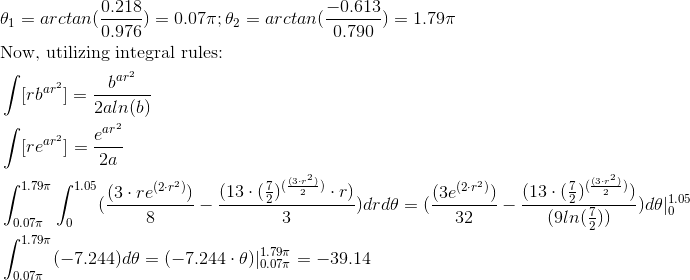Example Question #46 : Double Integration In Polar Coordinates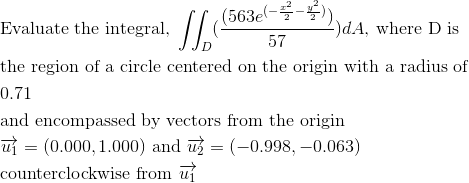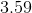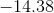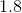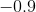Explanation: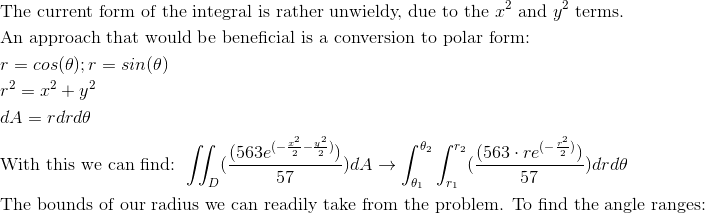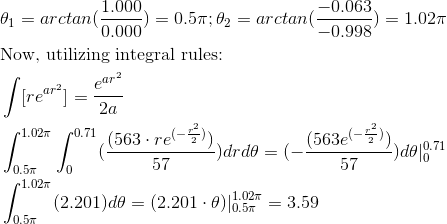Example Question #47 : Double Integration In Polar Coordinates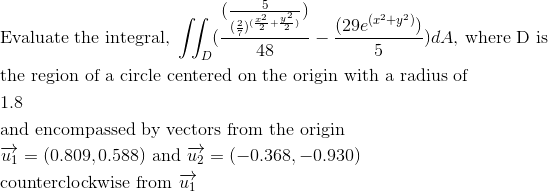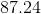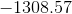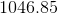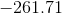Explanation: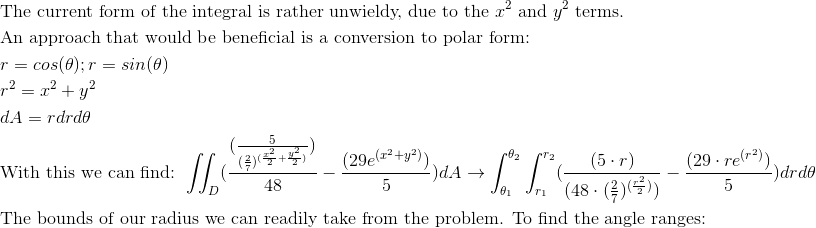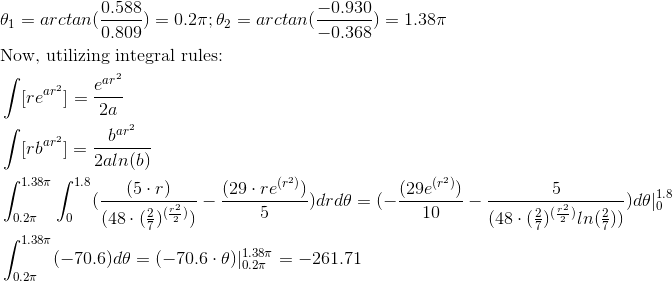Example Question #48 : Double Integration In Polar Coordinates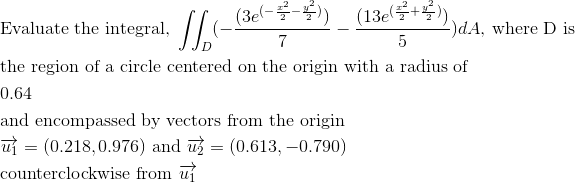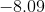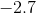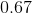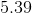Explanation: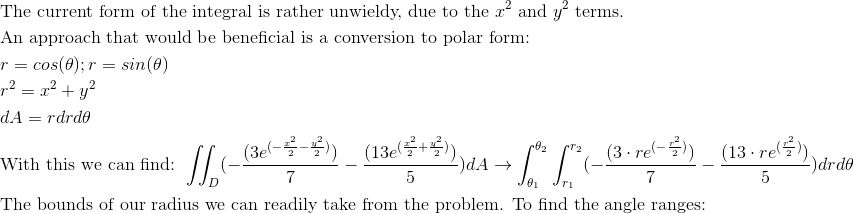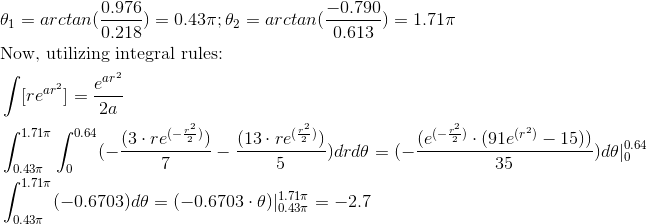Example Question #49 : Double Integration In Polar Coordinates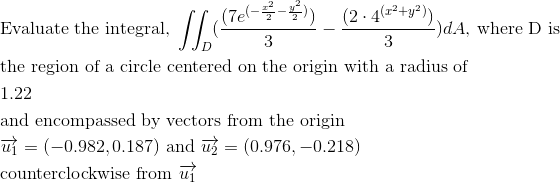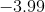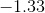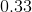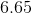Explanation: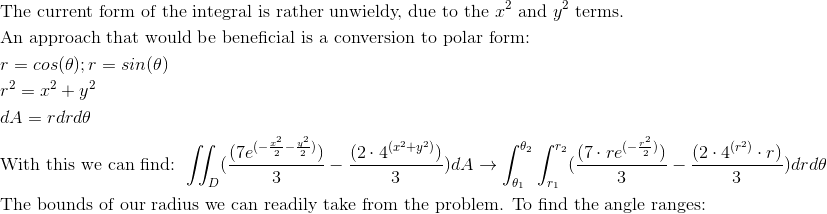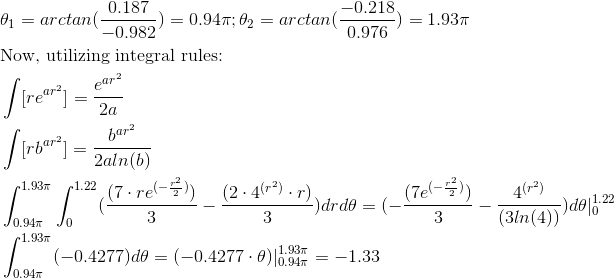Example Question #50 : Double Integration In Polar Coordinates